##用户名 Email 自动登录 找回密码 密码 立即注册
 搜索 热搜: 活动 交友 discuz

# caffe卷积操作的源码解析

213主题 1913积分1913发表于 2020-7-22 07:20:41 | 显示全部楼层 |阅读模式
 我认为这两位博主的理解是对的。 https://blog.csdn.net/Mrhiuser/article/details/52672824 https://blog.csdn.net/jiongnima/article/details/69736844 搜到我这篇博客的建议直接读他们的原作。我只做学习参考 caffe im2col详解 本文讲解的是caffe中卷积过程，众所周知caffe中卷积采用的是im2col和sgemm的方式。网上已经有很多的im2col的讲解。原本不打算写这篇文章（在此不得不吐槽下，网上有不少的讲解caffe im2col的过程的文章多是错的。一些文章和caffe的具体实现对不上，真不知道他们到底有没有看caffe代码的具体实现，正是因为那些文章，浪费了不少时间。） 本文举例讲解caffe中im2col 和 sgemm的具体过程。在此之前先说明下：caffe中的数据是行优先（row-major）存储的。 一：im2col 一个图像 input_num=1; 图像通道 input_channel=1; 图像高 input_h=4; 图像宽 input_w=4; kernel高 kernel_h=3; kernel宽 kernel_w=3; stride=1；pad=0； 卷积后，输出图像的计算公式： output_h=(input_h-kernel_h)/stride+1; output_w=(input_w-kernel_w)/stride+1; 如下图，（注：图像中数据不代表图像的颜色数值）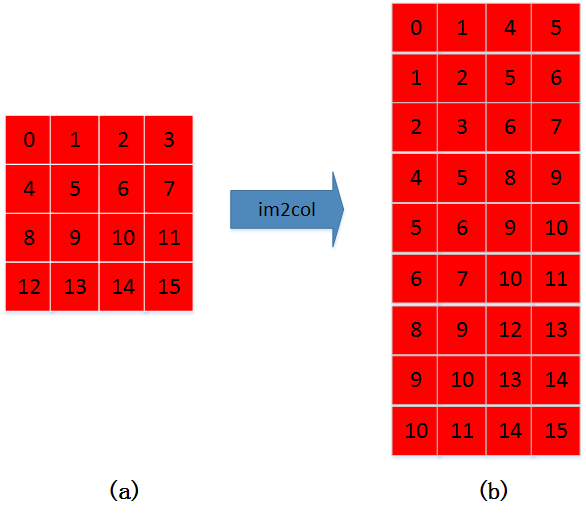原图（图a）按照从左到右、从上到下的过程，将(a)中大小为3*3（因为kernel大小为3*3）的矩阵拉成右图（图b）中的一列。具体过程如下图所示：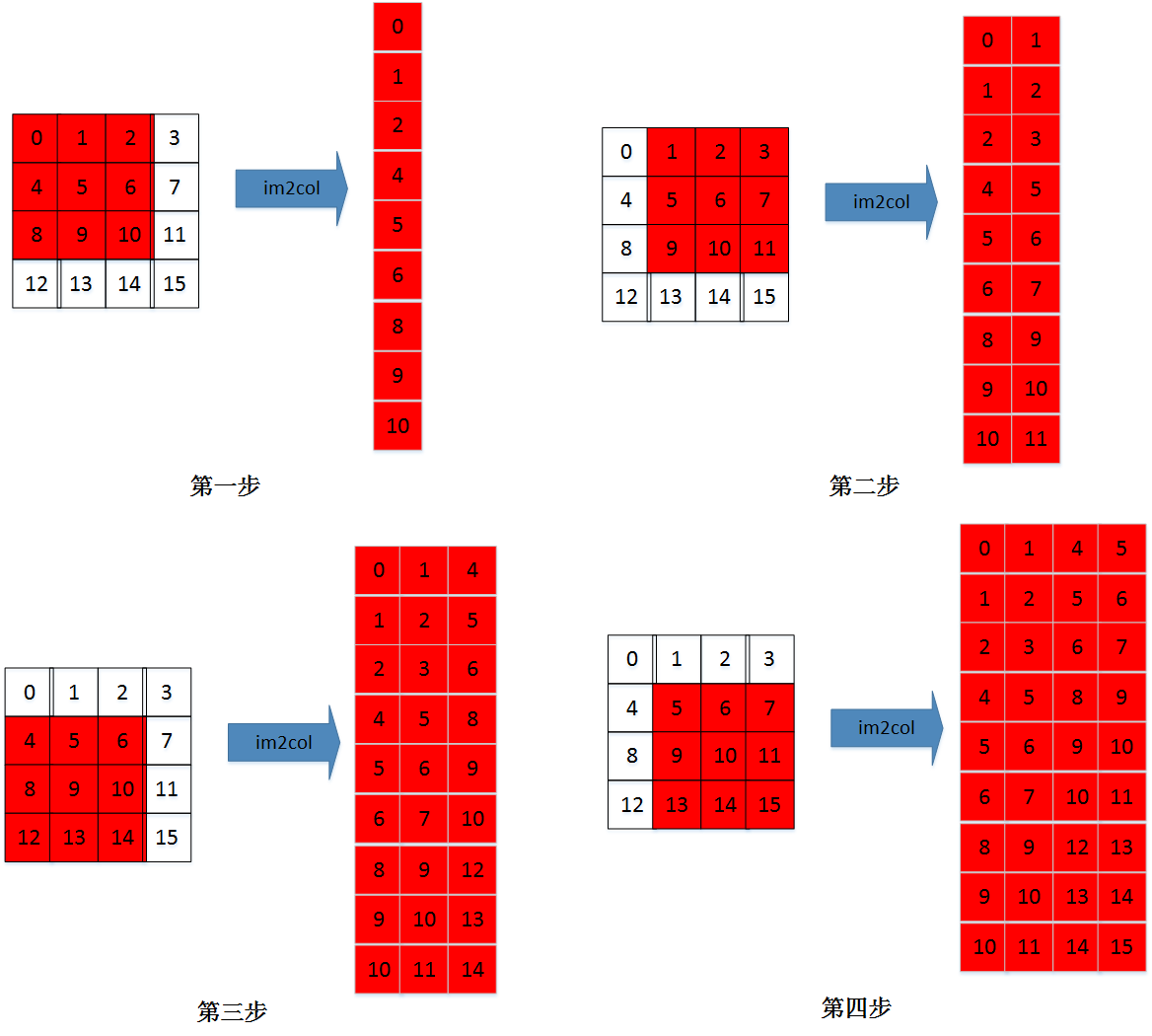二：多通道的im2col 假设有三个通道（R、G、B）图像通道 input_channel=3; 图像在内存中的存储是：首先是连续存储第一通道的数据，然后再存储第二通道的数据，最后存储第三通道的数据。如下图：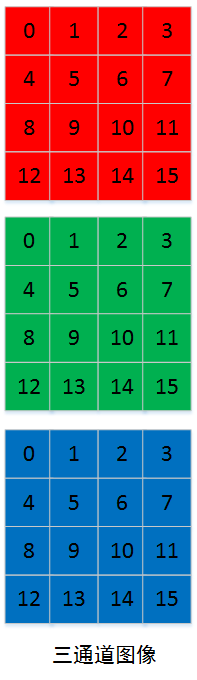多通道的im2col的过程，是首先im2col第一通道，然后在im2col第二通道，最后im2col第三通道。各通道im2col的数据在内存中也是连续存储的。如下图：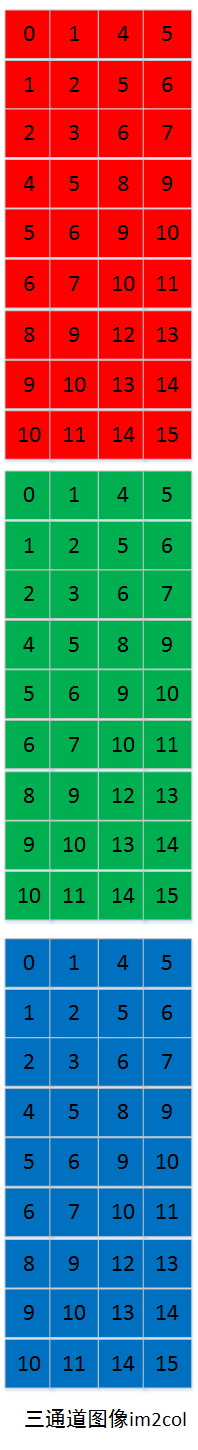三：kernel 图像的每个通道对应一个kernel通道，如下图（注：为计算简单，将kernel的值设置为1，同样此值不代表颜色数值。）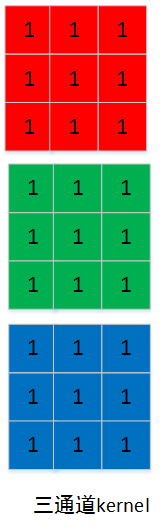kernel的通道数据在内存中也是连续存储的。所以上面的kernel图像也可以表示为下图：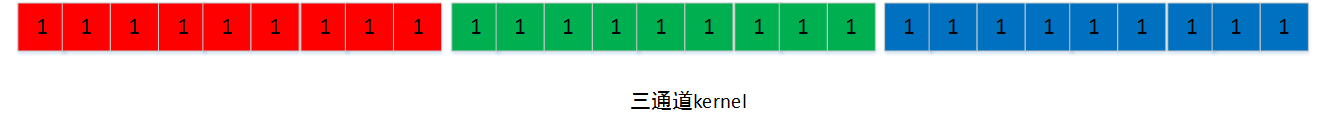三：矩阵乘 sgemm 在caffe中图像与kernel的矩阵乘，是kernel*img。即：在矩阵乘法中 M=1 ， N=output_h * output_w K=input_channels * kernel_h * kernel_w 如下图所示：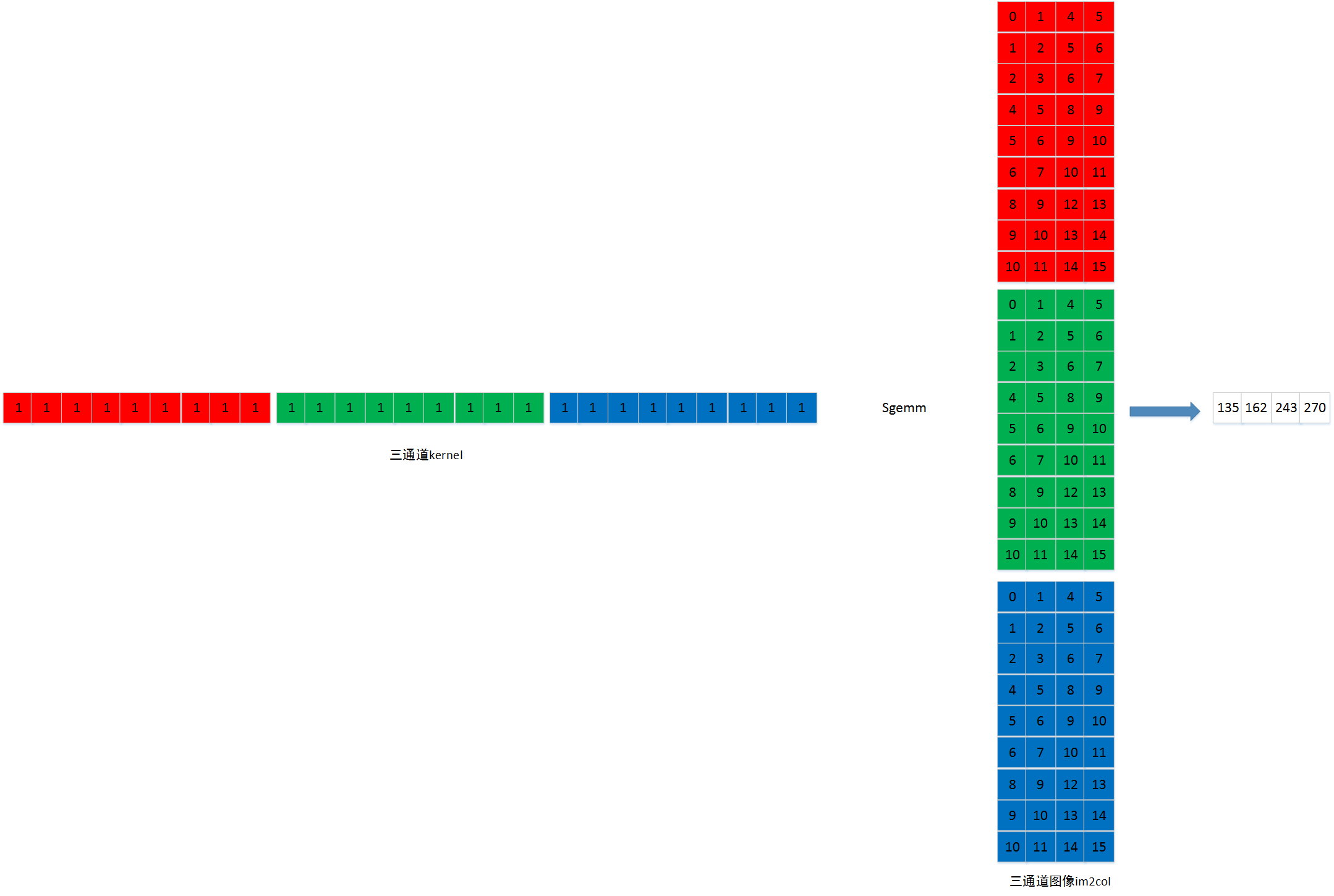图像数据是连续存储，因此输出图像也可以如下图所示【output_h * output_w】=【2*2】：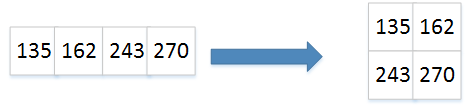四：多通道图像输出 在caffe中图像与kernel的矩阵乘中： M=output_channels ， N=output_h * output_w K=input_channels * kernel_h * kernel_w 如下图：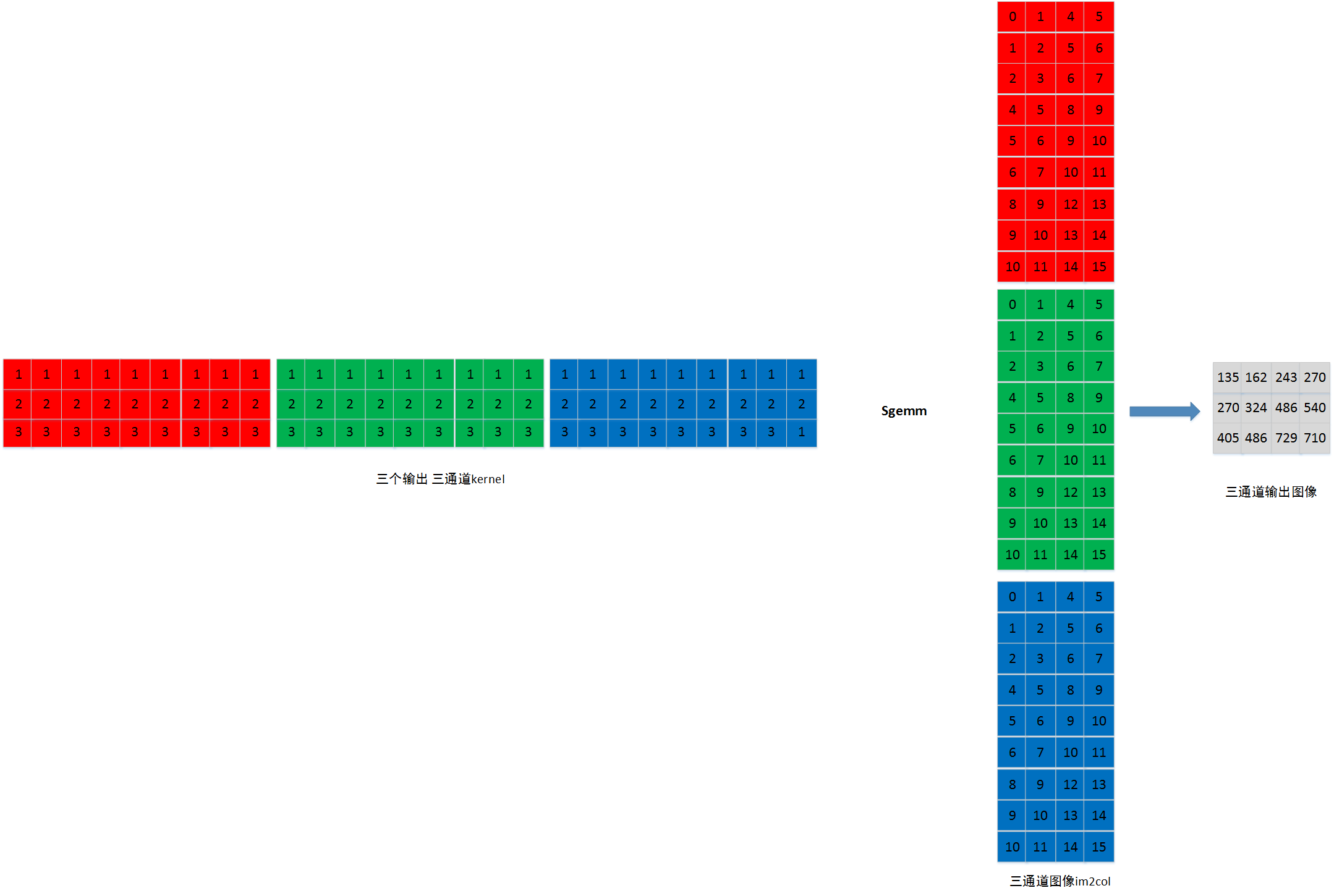同样，多个输出通道图像的数据是连续存储，因此输出图像也可以如下图所示【output_channels*output_h * output_w】=【3*2*2】,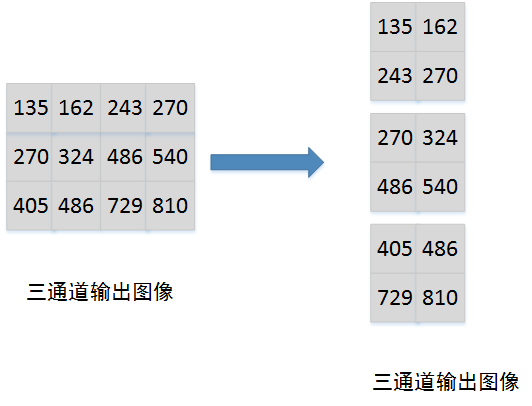(完) 在先前的两篇博客中，笔者详细解析了caffe卷积层的定义与实现，可是在conv_layer.cpp与base_conv_layer.cpp中，卷积操作的实现仍然被隐藏，通过im2col_cpu函数和caffe_cpu_gemm函数(后者实现矩阵乘法)实现，在此篇博客中，笔者旨在向大家展示，caffe的卷积操作是如何高效地通过向量转化和矩阵相乘完成的，并向大家解析caffe中的im2col操作，下面我们开始正文：   首先回顾一下卷积的实现理论细节，卷积核是一个小窗口(记录权重)，在输入图像上按步长滑动，每次滑动操作输入图像上的对应小窗区域，将卷积核中的各个权值与输入图像上对应小窗口中的各个值相乘，然后相加，并加上偏置得到输出特征图上的一个值，见下图(图片来自网络)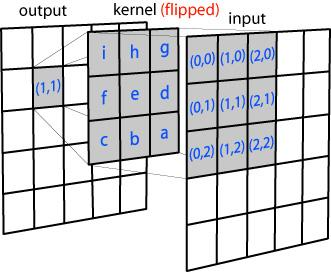请各位读者朋友思考，卷积核对输入特征图的每一次运算，是不是与两个向量的内积非常类似？这就意味着，卷积操作完全可以转化为矩阵的乘法来实现，事实上，caffe也是这么做的，卷积层对每一个blob的卷积操作可以看成权值矩阵与输入特征图转化成的矩阵进行相乘的运算，其中，权值矩阵的行数为一个卷积组的输出通道数，权值矩阵的列数为一个卷积组的输入通道数*卷积核高*卷积核宽；而输入特征图转化成的矩阵行数为一个卷积组的输入通道数*卷积核高*卷积核宽，列数为卷积层输出的单通道特征图高*卷积层输出的单通道特征图宽。   接下来笔者用式子清晰地表示一下caffe卷积操作的实现：卷积层输出 = 权值矩阵 * 输入特征图转化得到的矩阵权值矩阵尺度 = (卷积组输出通道数) * (卷积组输入通道数*卷积核高*卷积核宽)输入特征图转化得到的矩阵尺度 = (卷积组输入通道数*卷积核高*卷积核宽) * (卷积层输出单通道特征图高 * 卷积层输出单通道特征图宽)因此，卷积层输出尺度可以表示为卷积层输出尺度 = (卷积层输出通道数) * (卷积层输出单通道特征图高 * 卷积层输出单通道特征图宽)   到此是不是可以看到，卷积层输出尺度正好是理论上的卷积输出尺度。那么，在这个卷积乘法中，权值矩阵与输入特征图转化得到的矩阵是怎么得来的呢？这就是im2col.cpp中定义的了，也是本篇博客笔者解析的重点，下面笔者将以一张卷积层输入的单通道特征图为例，解析一下是通过怎样的操作生成相应的矩阵的。   首先给出is_a_ge_zero_and_a_lt_b函数的定义及注释： [cpp] view plain copy // Function uses casting from int to unsigned to compare if value of  // parameter a is greater or equal to zero and lower than value of  // parameter b. The b parameter is of type signed and is always positive,  // therefore its value is always lower than 0x800... where casting  // negative value of a parameter converts it to value higher than 0x800...  // The casting allows to use one condition instead of two.  inline bool is_a_ge_zero_and_a_lt_b(int a, int b) {//若a大于等于零或小于b，返回true，否则返回false    return static_cast(a) < static_cast(b);  }   // Function uses casting from int to unsigned to compare if value of // parameter a is greater or equal to zero and lower than value of // parameter b. The b parameter is of type signed and is always positive, // therefore its value is always lower than 0x800... where casting // negative value of a parameter converts it to value higher than 0x800... // The casting allows to use one condition instead of two. inline bool is_a_ge_zero_and_a_lt_b(int a, int b) {//若a大于等于零或小于b，返回true，否则返回false   return static_cast(a) < static_cast(b); } 该函数定义是：若a大于0且严格小于b，则返回真，否则返回假，该函数的作用是判断矩阵上某元的输出是否为pad的0。    然后给出im2col_cpu函数定义及注释： [cpp] view plain copy /*im2col_cpu将c个通道的卷积层输入图像转化为c个通道的矩阵，矩阵的行值为卷积核高*卷积核宽， 也就是说，矩阵的单列表征了卷积核操作一次处理的小窗口图像信息；而矩阵的列值为卷积层 输出单通道图像高*卷积层输出单通道图像宽，表示一共要处理多少个小窗口。 im2col_cpu接收13个参数，分别为输入数据指针(data_im)，卷积操作处理的一个卷积组的通道 数(channels)，输入图像的高(height)与宽(width)，原始卷积核的高(kernel_h)与宽(kernel_w)， 输入图像高(pad_h)与宽(pad_w)方向的pad，卷积操作高(stride_h)与宽(stride_w)方向的步长， 卷积核高(stride_h)与宽(stride_h)方向的扩展，输出矩阵数据指针(data_col)*/  template   void im2col_cpu(const Dtype* data_im, const int channels,      const int height, const int width, const int kernel_h, const int kernel_w,      const int pad_h, const int pad_w,      const int stride_h, const int stride_w,      const int dilation_h, const int dilation_w,      Dtype* data_col) {    const int output_h = (height + 2 * pad_h -      (dilation_h * (kernel_h - 1) + 1)) / stride_h + 1;//计算卷积层输出图像的高    const int output_w = (width + 2 * pad_w -      (dilation_w * (kernel_w - 1) + 1)) / stride_w + 1;//计算卷积层输出图像的宽    const int channel_size = height * width;//计算卷积层输入单通道图像的数据容量    /*第一个for循环表示输出的矩阵通道数和卷积层输入图像通道是一样的，每次处理一个输入通道的信息*/    for (int channel = channels; channel--; data_im += channel_size) {      /*第二个和第三个for循环表示了输出单通道矩阵的某一列，同时体现了输出单通道矩阵的行数*/      for (int kernel_row = 0; kernel_row < kernel_h; kernel_row++) {        for (int kernel_col = 0; kernel_col < kernel_w; kernel_col++) {          int input_row = -pad_h + kernel_row * dilation_h;//在这里找到卷积核中的某一行在输入图像中的第一个操作区域的行索引          /*第四个和第五个for循环表示了输出单通道矩阵的某一行，同时体现了输出单通道矩阵的列数*/          for (int output_rows = output_h; output_rows; output_rows--) {            if (!is_a_ge_zero_and_a_lt_b(input_row, height)) {//如果计算得到的输入图像的行值索引小于零或者大于输入图像的高(该行为pad)              for (int output_cols = output_w; output_cols; output_cols--) {                *(data_col++) = 0;//那么将该行在输出的矩阵上的位置置为0              }            } else {              int input_col = -pad_w + kernel_col * dilation_w;//在这里找到卷积核中的某一列在输入图像中的第一个操作区域的列索引              for (int output_col = output_w; output_col; output_col--) {                if (is_a_ge_zero_and_a_lt_b(input_col, width)) {//如果计算得到的输入图像的列值索引大于等于于零或者小于输入图像的宽(该列不是pad)                  *(data_col++) = data_im[input_row * width + input_col];//将输入特征图上对应的区域放到输出矩阵上                } else {//否则，计算得到的输入图像的列值索引小于零或者大于输入图像的宽(该列为pad)                  *(data_col++) = 0;//将该行该列在输出矩阵上的位置置为0                }                input_col += stride_w;//按照宽方向步长遍历卷积核上固定列在输入图像上滑动操作的区域              }            }            input_row += stride_h;//按照高方向步长遍历卷积核上固定行在输入图像上滑动操作的区域          }        }      }    }  }   /*im2col_cpu将c个通道的卷积层输入图像转化为c个通道的矩阵，矩阵的行值为卷积核高*卷积核宽， 也就是说，矩阵的单列表征了卷积核操作一次处理的小窗口图像信息；而矩阵的列值为卷积层 输出单通道图像高*卷积层输出单通道图像宽，表示一共要处理多少个小窗口。 im2col_cpu接收13个参数，分别为输入数据指针(data_im)，卷积操作处理的一个卷积组的通道 数(channels)，输入图像的高(height)与宽(width)，原始卷积核的高(kernel_h)与宽(kernel_w)， 输入图像高(pad_h)与宽(pad_w)方向的pad，卷积操作高(stride_h)与宽(stride_w)方向的步长， 卷积核高(stride_h)与宽(stride_h)方向的扩展，输出矩阵数据指针(data_col)*/ template void im2col_cpu(const Dtype* data_im, const int channels,     const int height, const int width, const int kernel_h, const int kernel_w,     const int pad_h, const int pad_w,     const int stride_h, const int stride_w,     const int dilation_h, const int dilation_w,     Dtype* data_col) {   const int output_h = (height + 2 * pad_h -     (dilation_h * (kernel_h - 1) + 1)) / stride_h + 1;//计算卷积层输出图像的高   const int output_w = (width + 2 * pad_w -     (dilation_w * (kernel_w - 1) + 1)) / stride_w + 1;//计算卷积层输出图像的宽   const int channel_size = height * width;//计算卷积层输入单通道图像的数据容量   /*第一个for循环表示输出的矩阵通道数和卷积层输入图像通道是一样的，每次处理一个输入通道的信息*/   for (int channel = channels; channel--; data_im += channel_size) {         /*第二个和第三个for循环表示了输出单通道矩阵的某一列，同时体现了输出单通道矩阵的行数*/     for (int kernel_row = 0; kernel_row < kernel_h; kernel_row++) {       for (int kernel_col = 0; kernel_col < kernel_w; kernel_col++) {         int input_row = -pad_h + kernel_row * dilation_h;//在这里找到卷积核中的某一行在输入图像中的第一个操作区域的行索引                 /*第四个和第五个for循环表示了输出单通道矩阵的某一行，同时体现了输出单通道矩阵的列数*/         for (int output_rows = output_h; output_rows; output_rows--) {           if (!is_a_ge_zero_and_a_lt_b(input_row, height)) {//如果计算得到的输入图像的行值索引小于零或者大于输入图像的高(该行为pad)             for (int output_cols = output_w; output_cols; output_cols--) {               *(data_col++) = 0;//那么将该行在输出的矩阵上的位置置为0             }           } else {             int input_col = -pad_w + kernel_col * dilation_w;//在这里找到卷积核中的某一列在输入图像中的第一个操作区域的列索引             for (int output_col = output_w; output_col; output_col--) {               if (is_a_ge_zero_and_a_lt_b(input_col, width)) {//如果计算得到的输入图像的列值索引大于等于于零或者小于输入图像的宽(该列不是pad)                 *(data_col++) = data_im[input_row * width + input_col];//将输入特征图上对应的区域放到输出矩阵上               } else {//否则，计算得到的输入图像的列值索引小于零或者大于输入图像的宽(该列为pad)                 *(data_col++) = 0;//将该行该列在输出矩阵上的位置置为0               }               input_col += stride_w;//按照宽方向步长遍历卷积核上固定列在输入图像上滑动操作的区域             }           }           input_row += stride_h;//按照高方向步长遍历卷积核上固定行在输入图像上滑动操作的区域         }       }     }   } }    im2col_cpu函数将卷积层输入转化为矩阵相乘的右元，核心是5个for循环，首先第一个for循环表示按照输入的通道数逐个处理卷积层输入的特征图，下面笔者将用图示表示剩余的四个for循环操作，向读者朋友们展示卷积层输入的单通道特征图是通过怎样的方式转化为一个矩阵。在这里我们假设，卷积层输入单通道特征图原大小为5*5，高和宽方向的pad为1，高和宽方向步长为2，卷积核不进行扩展。   我们先计算一下，卷积层输入单通道特征图转化得到的矩阵的尺度，矩阵的行数应该为卷积核高*卷积核宽，即为9，列数应该为卷积层输出特征图高(output_h)*卷积层输出特征图宽(output_w)，也为9，那么，im2col算法起始由下图开始：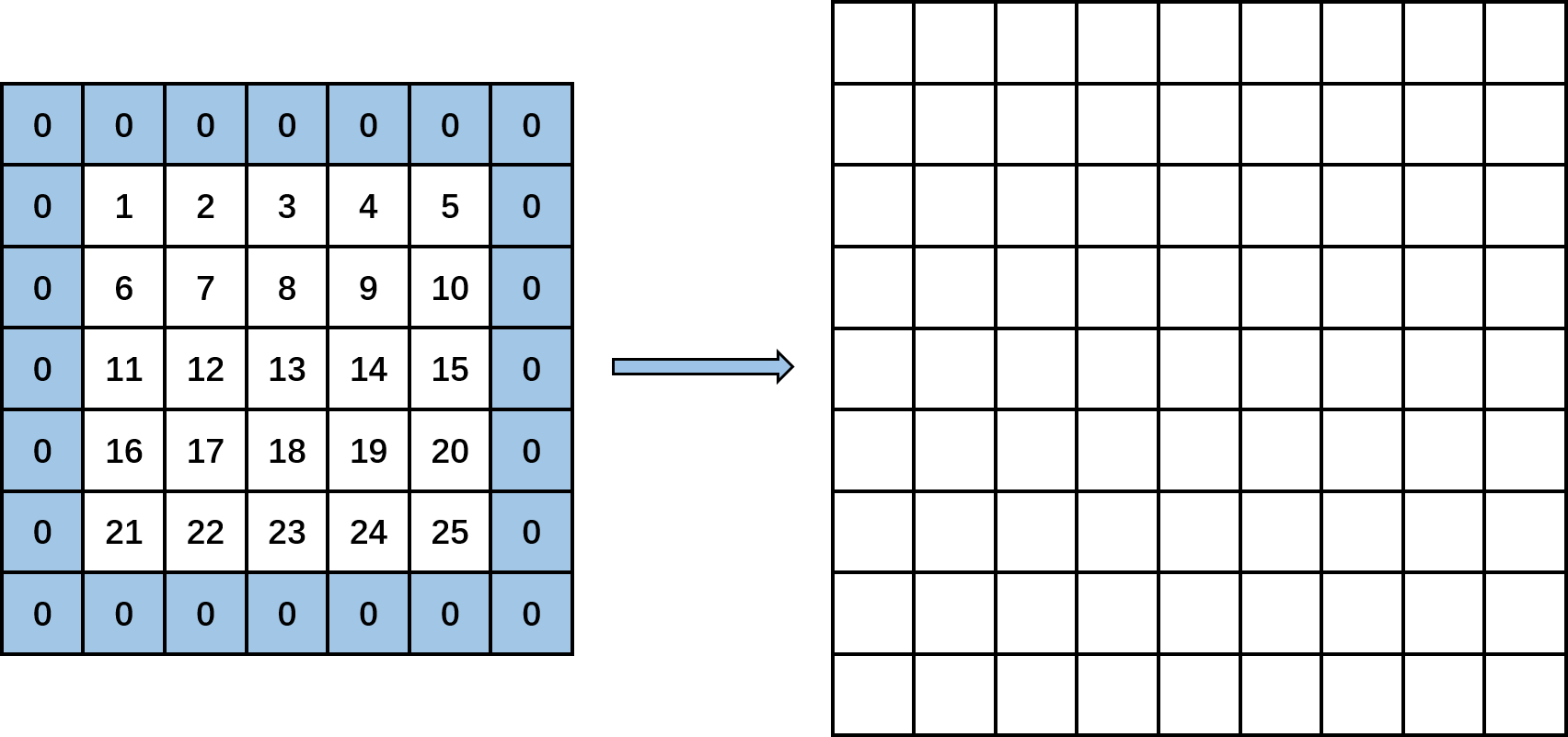首先kernel_row为0，kernel_col也为0。按照input_row = -pad_h + kernel_row * dilation_h计算input_row的值，在这里，pad_h为1，kernel_row为0，dilation_h为1，计算出input_row为-1，此时output_row为3，满足函数中的第一个if条件，那么在输出图像上先置output_w个零，因为output_w为3，因此得到下图：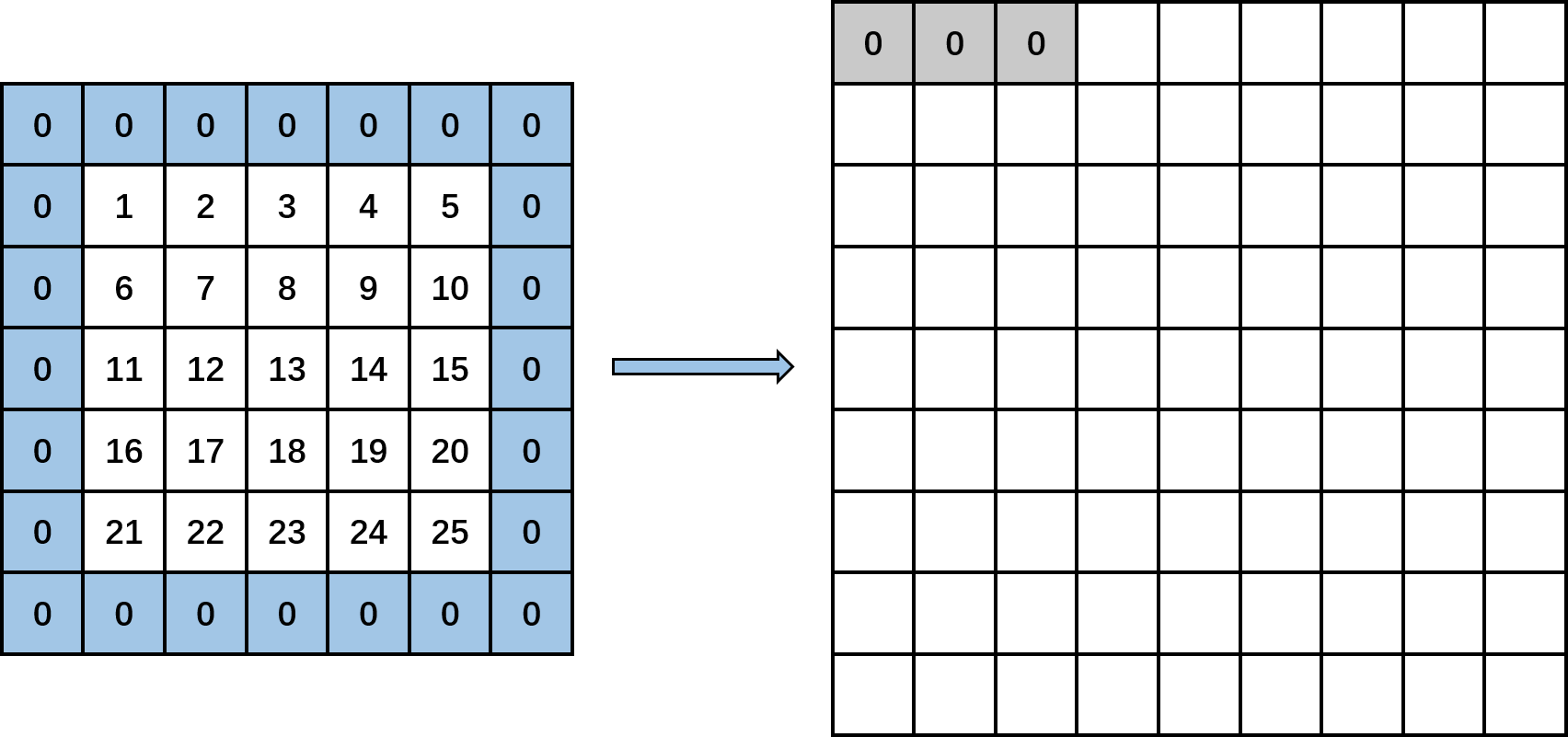然后input_row加上步长2，由-1变成1，此时output_rows为2，计算input_col等于-1，此时执行input_col定义下面的for循环，得到3个值：依次往目标矩阵中填入0，data_im[1*5+1]和data_im[1*5+3]，即填入0,7和9。得到下图：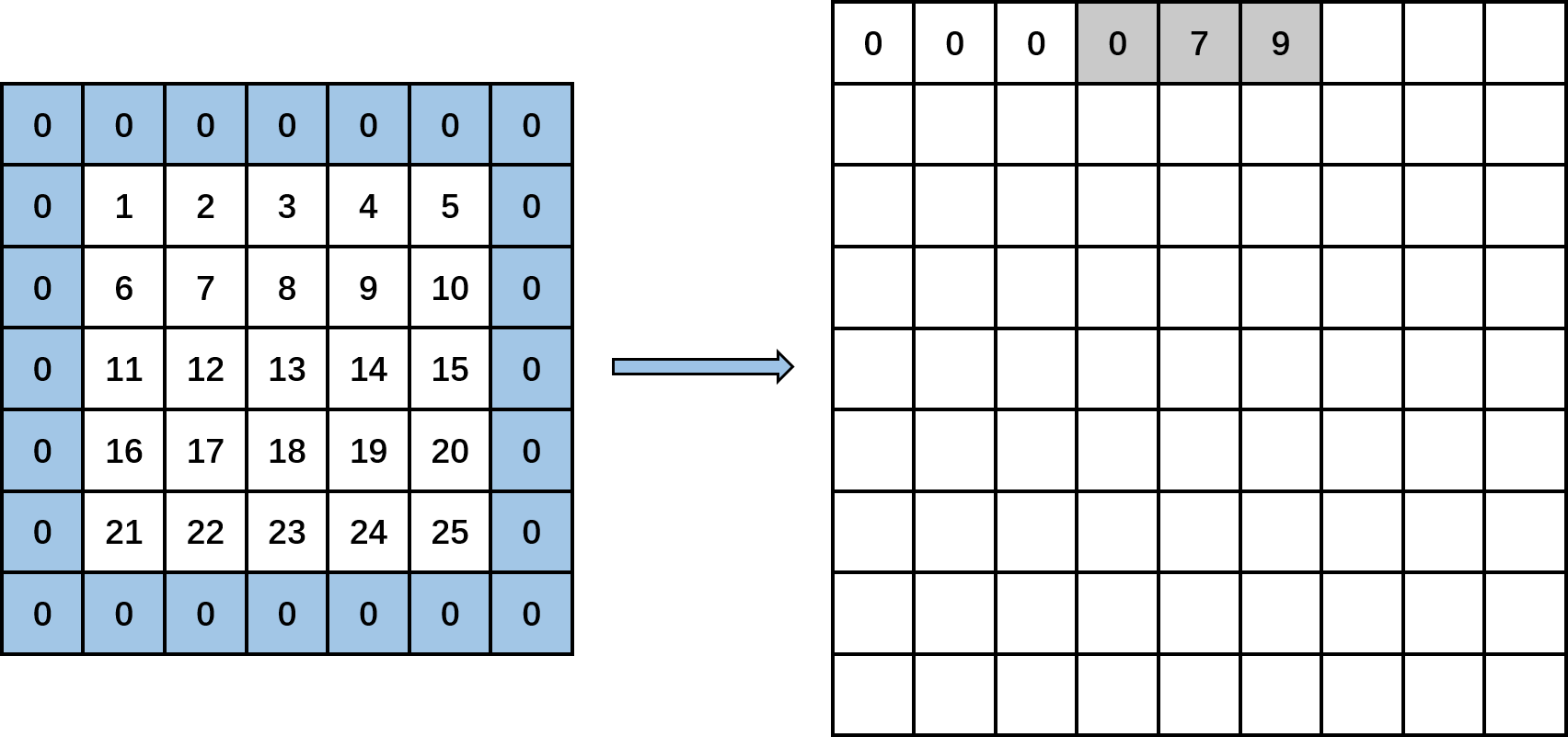再接着执行，此时input_row再加上2变为3，此时output_rows变为1，计算input_col等于-1，执行input_col定义下面的for循环，得到3个值，分别为0，data_im[3*5+1]和data_im[3*5+3]，即填入0,17和19。得到下图：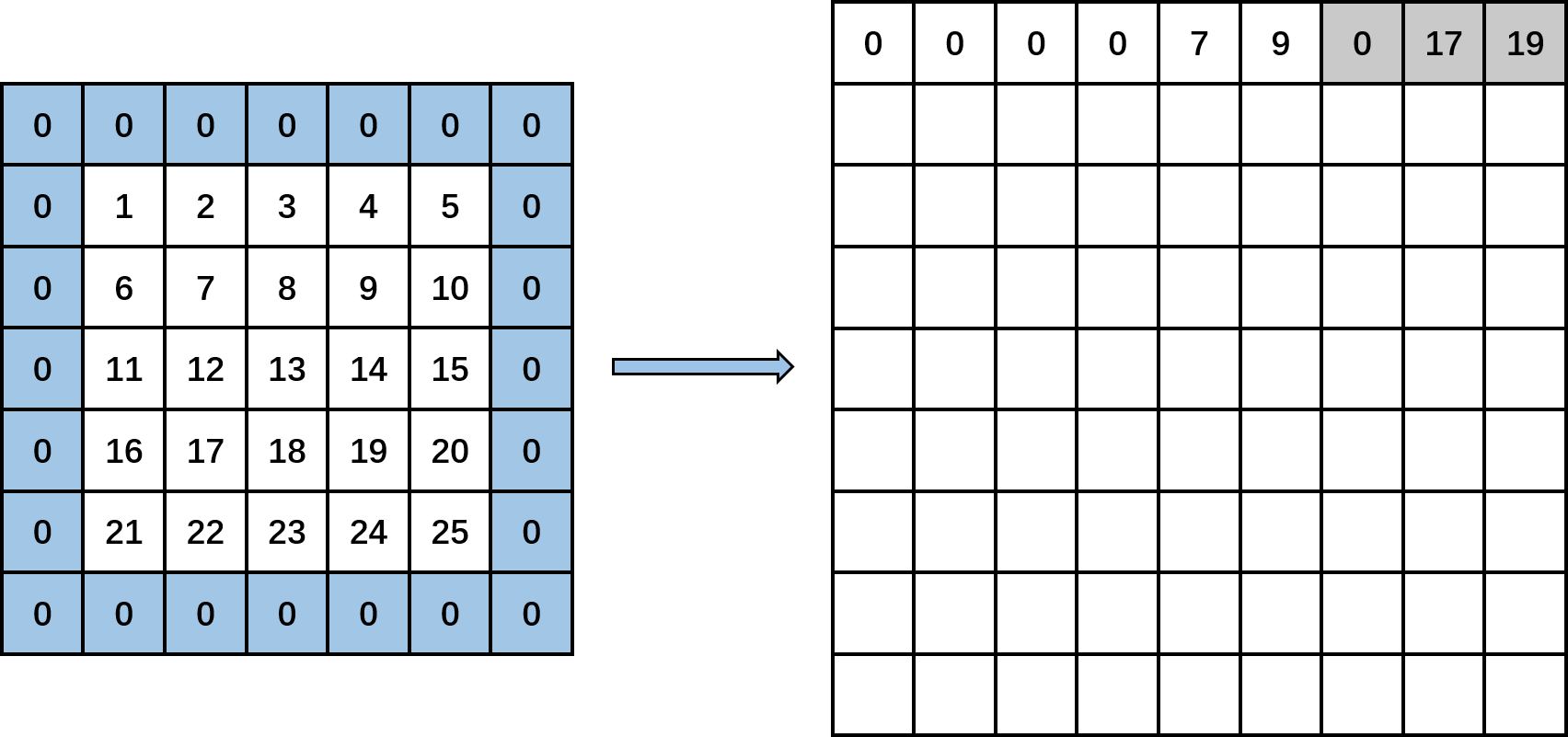接着，kernel_col变成1，此时kernel_row为0，kernel_col为1。计算input_row又变成-1，第一个if条件成立，那么，再在输出矩阵上输出3个0。然后，input_row变成1，input_col分别为0(-1+1)，2(-1+1+2)和4(-1+1+2+2)时，输出矩阵上分别输出data_im[1*5+0]，data[1*5+2]，data[1*5+4]，即分别填入6,8,10。然后，input_row变成3，input_col分别为0，2，4时，输出矩阵上分别输出data_im[3*5+0]，data[3*5+2]，data[3*5+4]，即分别输出16,18,20。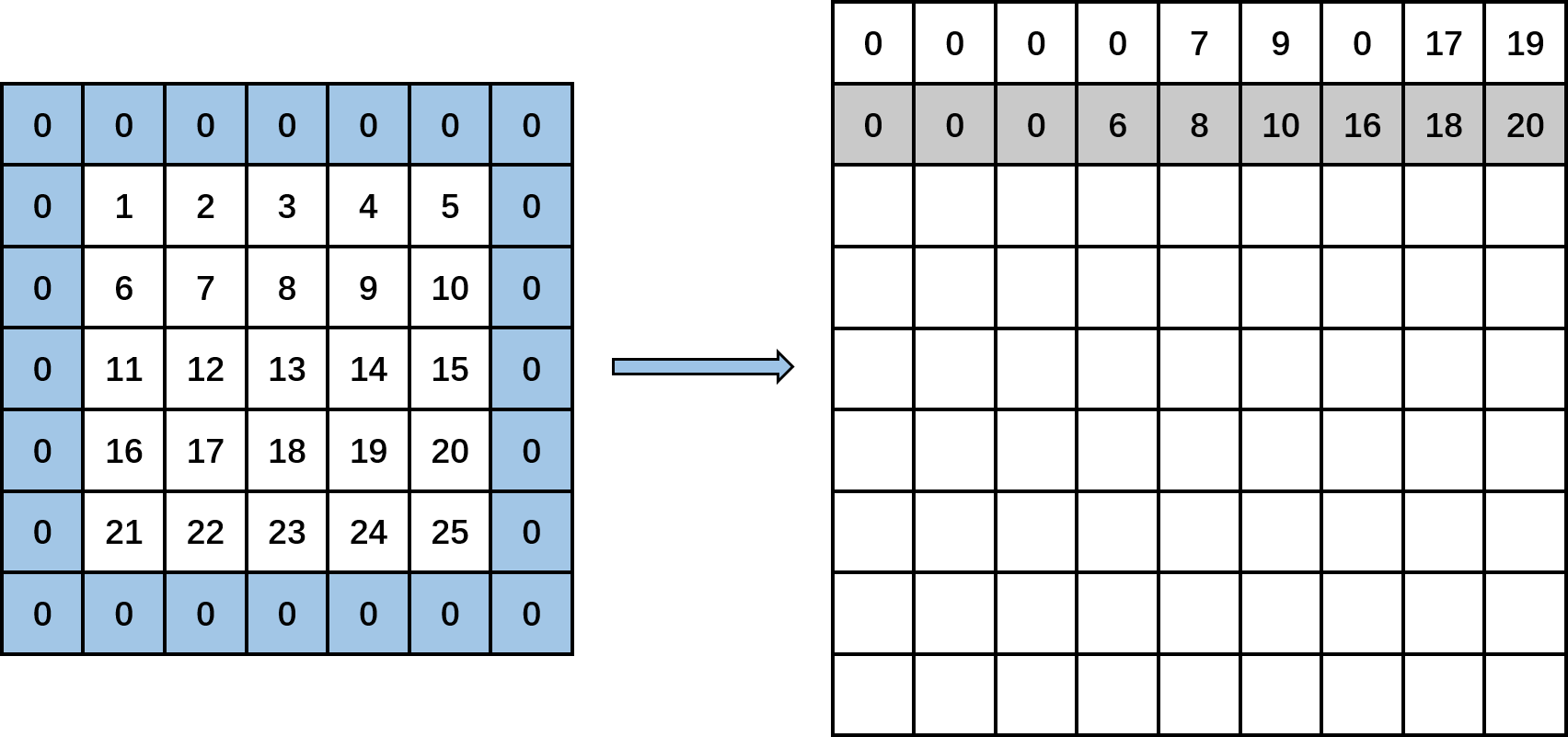然后，kernel_col变成2，此时kernel_row为0，kernel_col为2。计算input_row又变成-1，第一个if条件成立，那么，再在输出矩阵上输出3个0。然后，input_row变成1，input_col分别为1(-1+2)，3(-1+2+2)和5(-1+2+2+2)时，输出矩阵上分别输出data_im[1*5+1]，data[1*5+3]，0，即分别填入7,9,0。然后，input_row变成3，input_col分别为1，3，5时，输出矩阵上分别输出data_im[3*5+0]，data[3*5+2]，0，即分别输出17,19,0。见下图：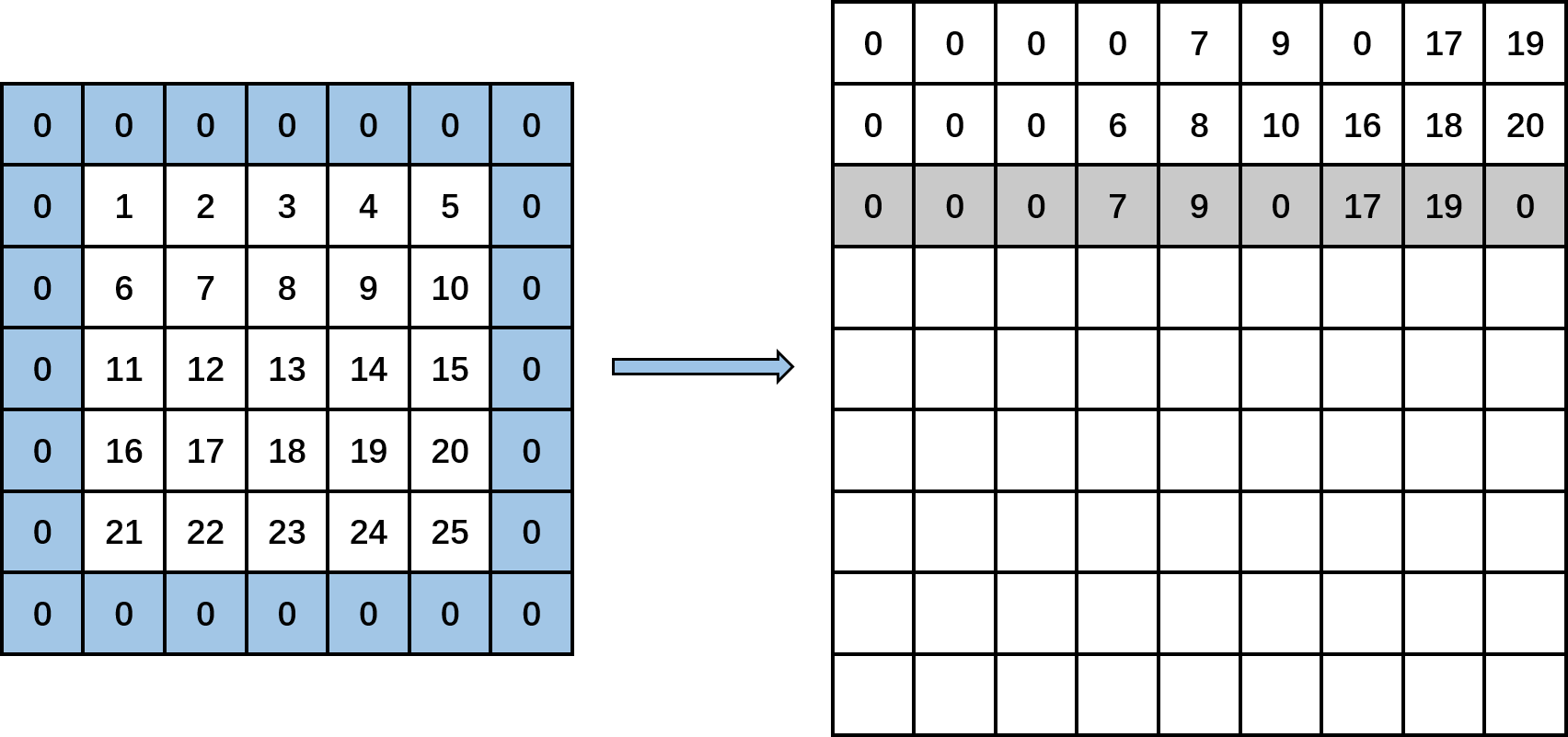接着，kernel_row变成1，kernel_col变成0。计算input_row又变成0，input_col分别为-1(-1+0)，1(-1+0+2)和3(-1+0+2+2)，输出矩阵上分别输出0，data[0*5+1]，data[0*5+3]，即分别填入0,2,4。然后，input_row变成2，input_col分别为-1，1和3时，输出矩阵上分别输出0，data[2*5+1]，data[2*5+3]，即分别填入0,12,14。然后，input_row变成4，input_col分别为-1，1，3时，输出矩阵上分别输出0，data[4*5+1]，data[4*5+3]，即分别输出0,22,24。见下图：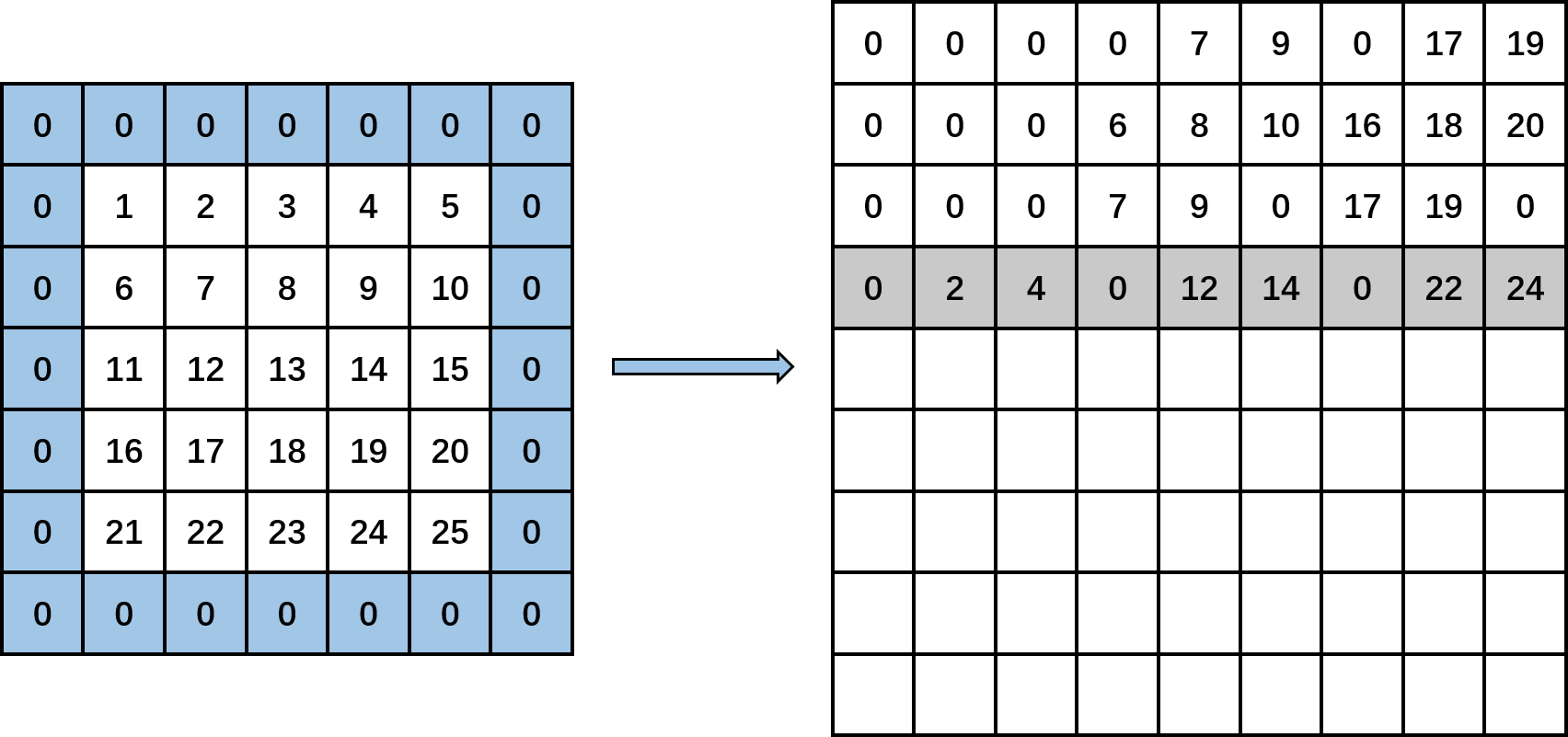然后，kernel_row为1，kernel_col变成1。计算input_row为0，input_col分别为0(-1+1)，2(-1+1+2)和4(-1+1+2+2)，输出矩阵上分别输出data[0*5+0]，data[0*5+2]，data[0*5+4]，即分别填入1,3,5。然后，input_row变成2，input_col分别为0，2和4时，输出矩阵上分别输出data[2*5+0]，data[2*5+2]，data[2*5+4]，即分别填入11,13,15。然后，input_row变成4，input_col分别为0，2，4时，输出矩阵上分别输出data[4*5+0]，data[4*5+2]，data[4*5+4]，即分别输出21,23,25。见下图：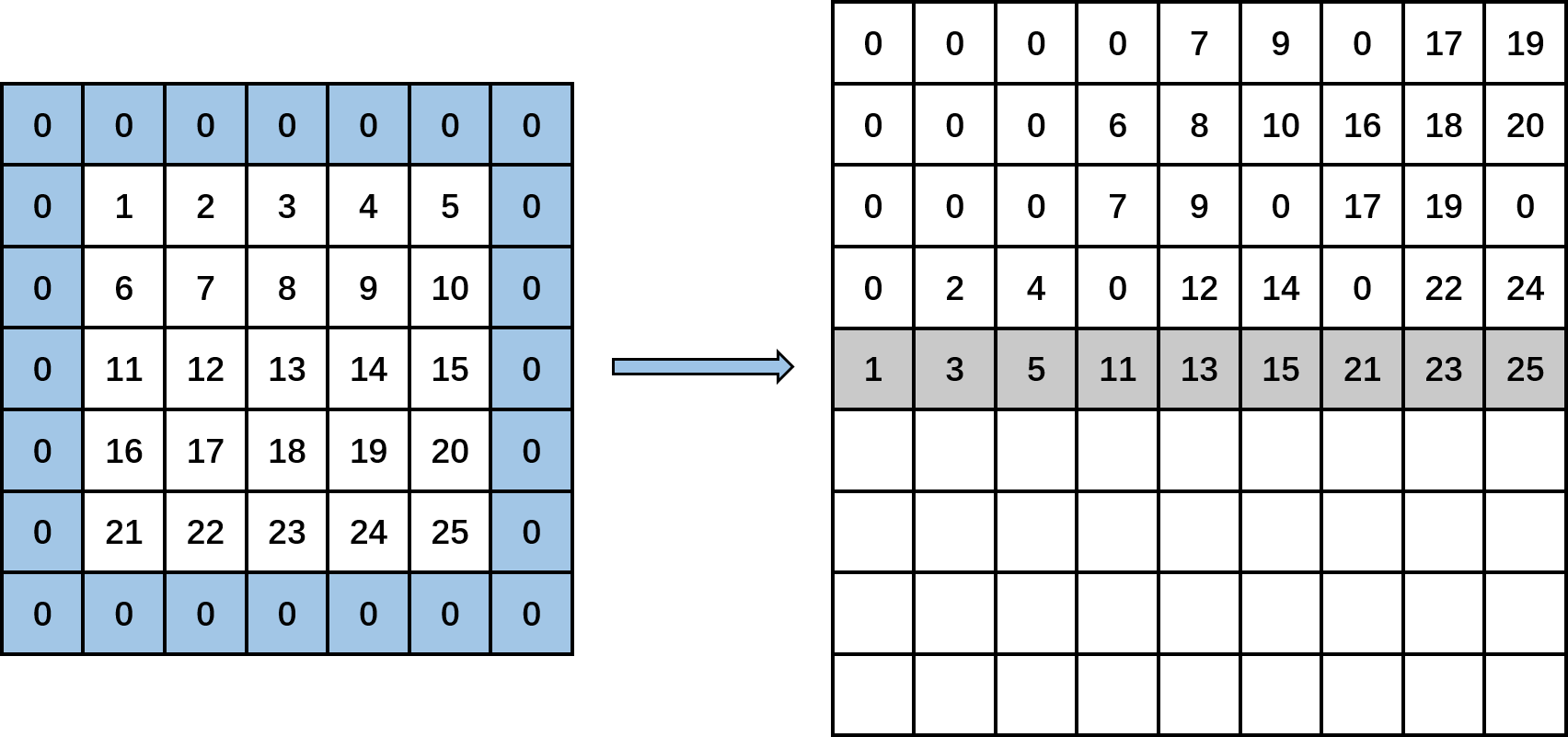然后，kernel_row为1，kernel_col变成2。计算input_row为0，input_col分别为1(-1+2)，3(-1+2+2)和5(-1+2+2+2)，输出矩阵上分别输出data[0*5+1]，data[0*5+3]，0，即分别填入2,4,0。然后，input_row变成2，input_col分别为1，3和5时，输出矩阵上分别输出data[2*5+1]，data[2*5+3]，0，即分别填入12,14,0。然后，input_row变成4，input_col分别为1，3，5时，输出矩阵上分别输出data[4*5+1]，data[4*5+3]，0，即分别输出22,24,0。见下图：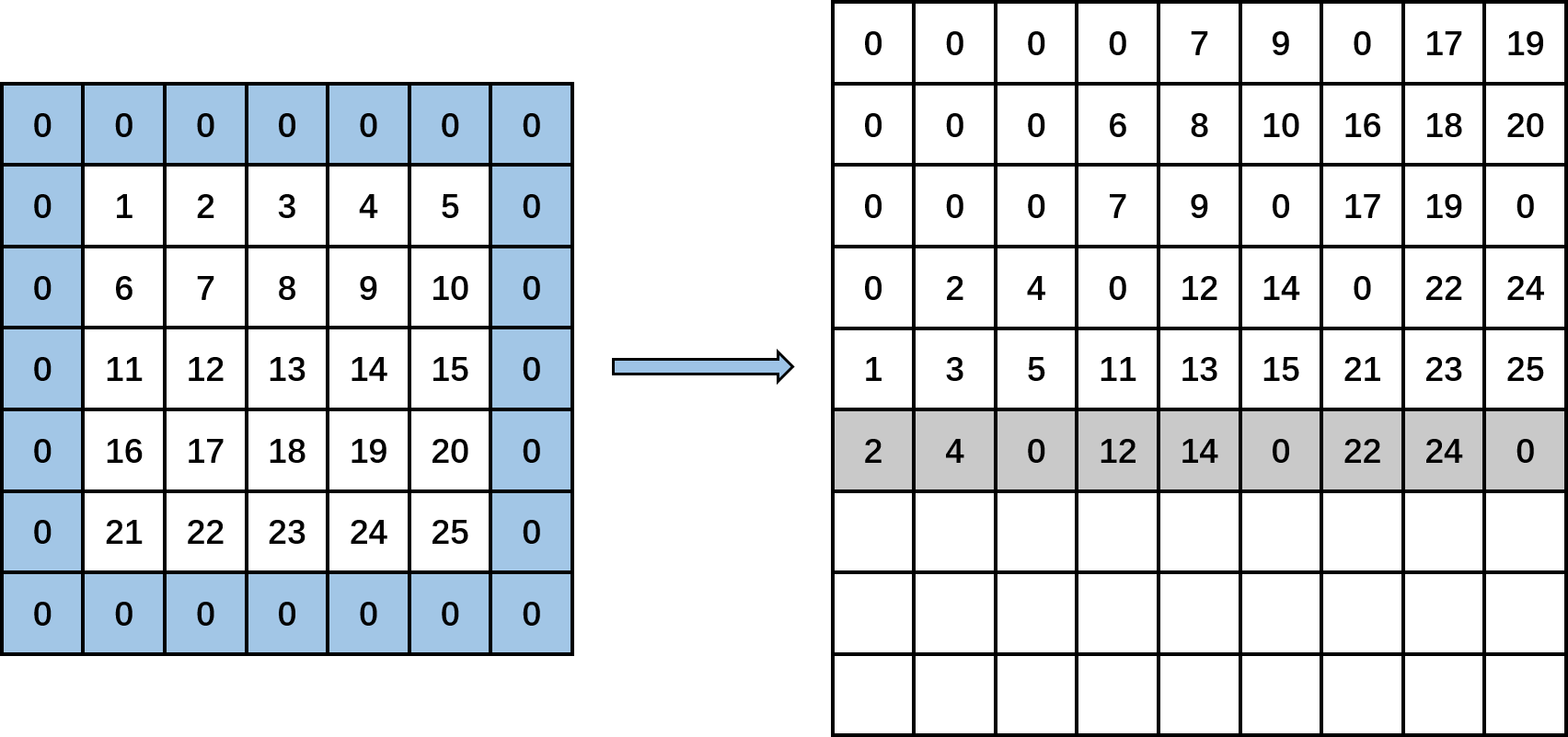接着，kernel_row变成2，kernel_col变成0。计算input_row为1，input_col分别为-1(-1+0)，1(-1+0+2)和3(-1+0+2+2)，输出矩阵上分别输出0，data[1*5+1]，data[1*5+3]，即分别填入0,7,9。然后，input_row变成3，input_col分别为-1，1和3时，输出矩阵上分别输出0，data[3*5+1]，data[3*5+3]，即分别填入0,17,19。然后，input_row变成5，满足第一个if条件，直接输出三个0。见下图：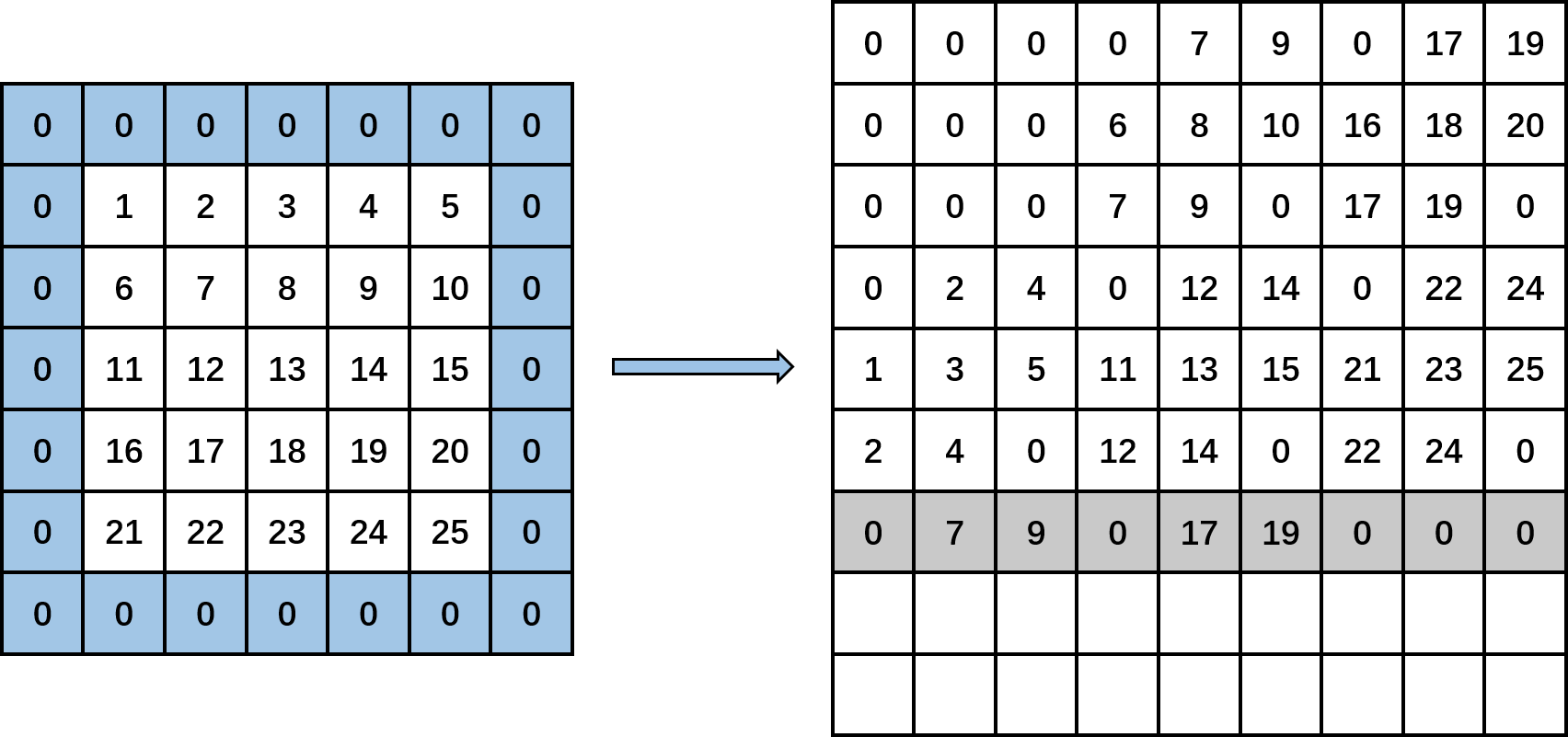然后，kernel_row为2，kernel_col变成1。计算input_row为1，input_col分别为0(-1+1)，2(-1+1+2)和4(-1+1+2+2)，输出矩阵上分别输出data[1*5+0]，data[1*5+2]，data[1*5+4]，即分别填入6,8,10。然后，input_row变成3，input_col分别为0，2和4时，输出矩阵上分别输出data[3*5+0]，data[3*5+2]，data[3*5+4]，即分别填入16,18,20。然后，input_row变成5，满足第一个if条件，直接输出三个0。见下图：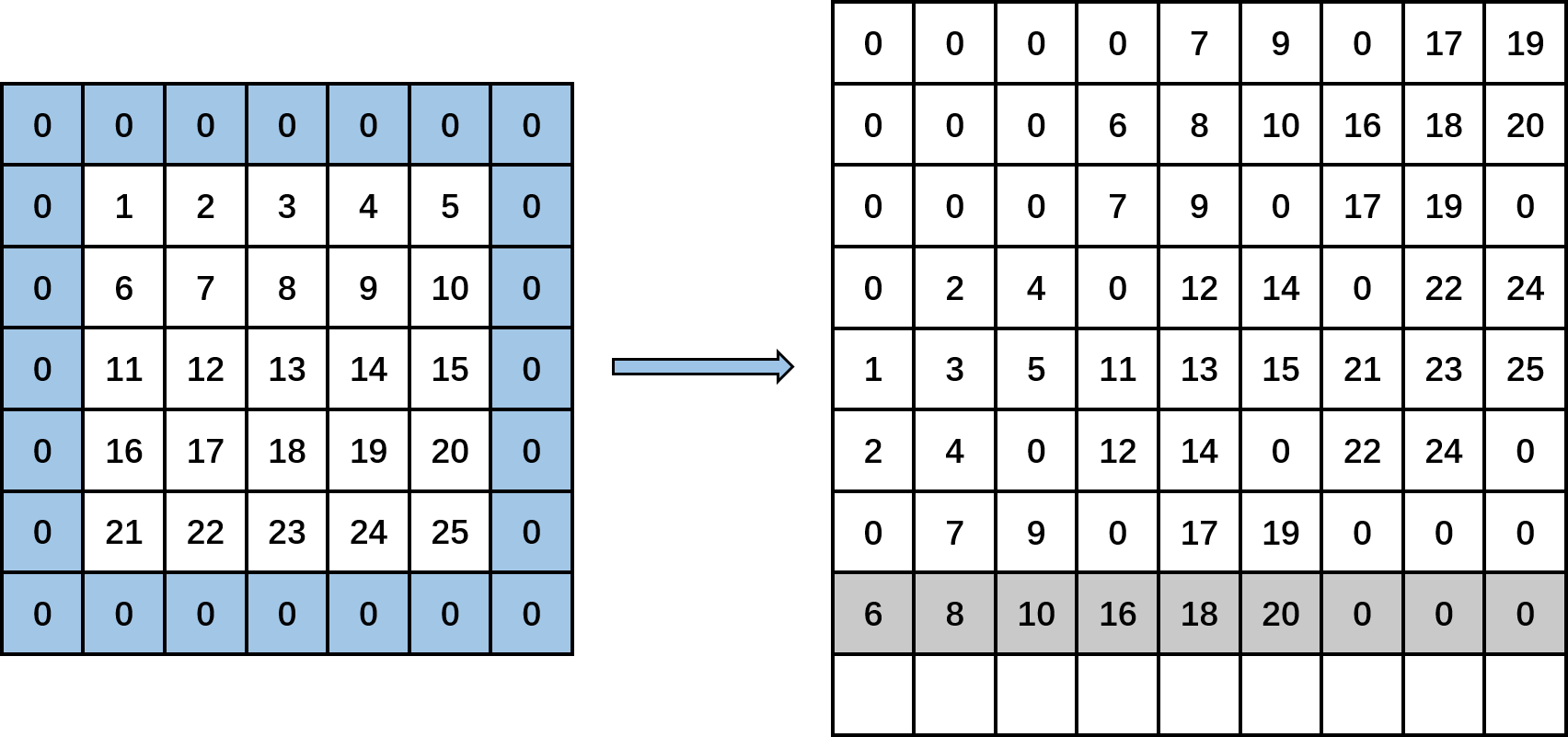最后，kernel_row为2，kernel_col变成2。计算input_row为1，input_col分别为1(-1+2)，3(-1+2+2)和5(-1+2+2+2)，输出矩阵上分别输出data[1*5+1]，data[1*5+3]，0，即分别填入7,9,0。然后，input_row变成3，input_col分别为1，3和5时，输出矩阵上分别输出data[3*5+1]，data[3*5+3]，0，即分别填入17,19,0。然后，input_row变成5，满足第一个if条件，直接输出三个0。见下图：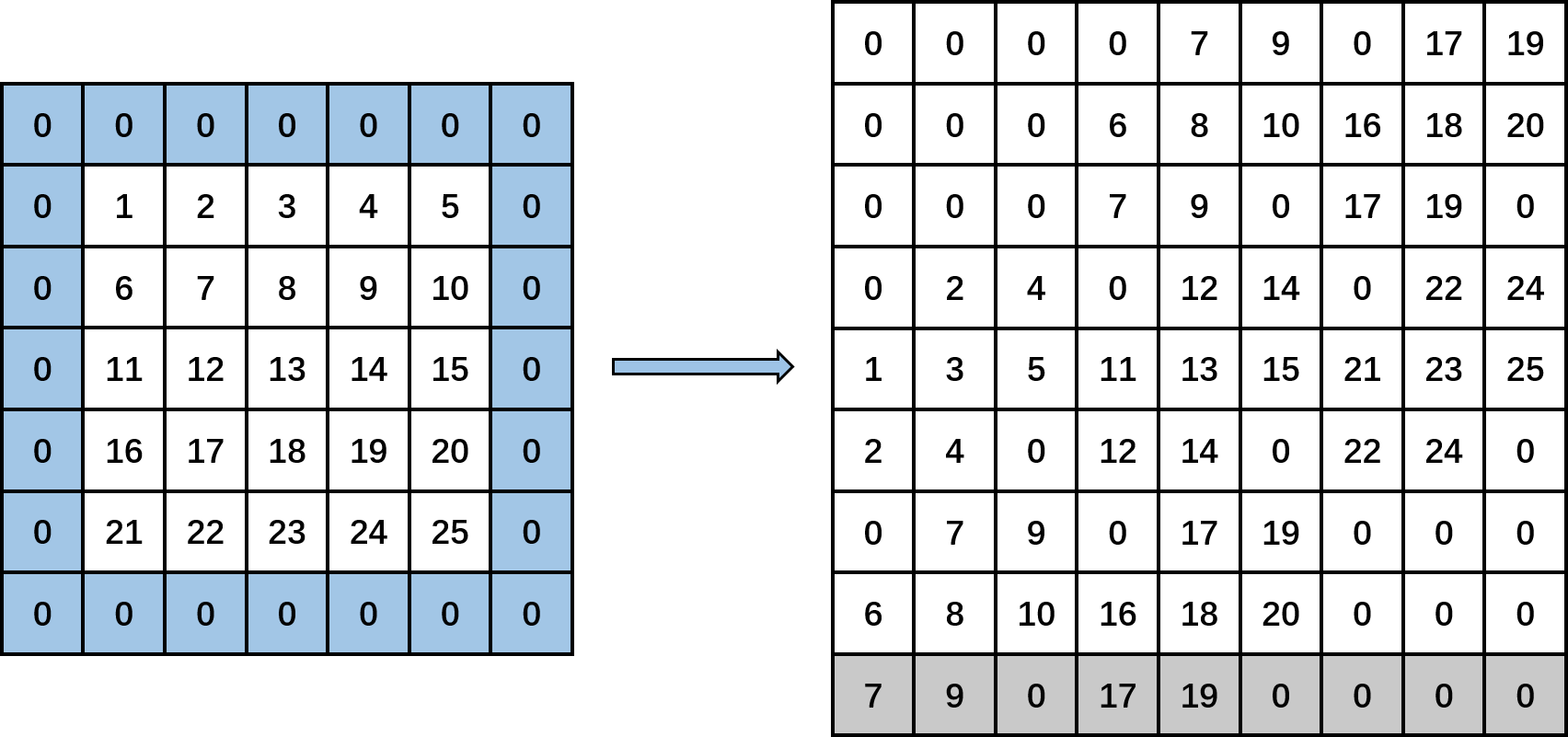到此卷积层单通道输入特征图就转化成了一个矩阵，请读者朋友们仔细看看，矩阵的各列是不是卷积核操作的各小窗口呢？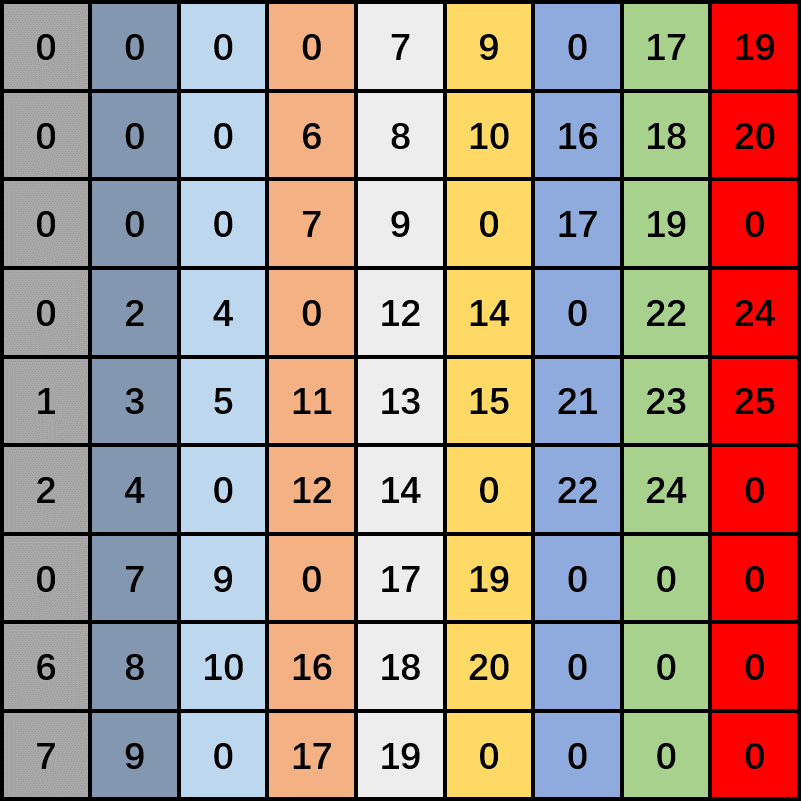笔者还想提醒大家的是，注意卷积中的zero-pad操作的实现，并不是真正在原始输入特征图周围添加0，而是在特征图转化得到的矩阵上的对应位置添加0。   而im2col_cpu函数功能的相反方向的实现则有由col2im_cpu函数完成，笔者依旧把该函数的代码注释放在下面： [cpp] view plain copy /*col2im_cpu为im2col_cpu的逆操作接收13个参数，分别为输入矩阵数据指针(data_col)，卷积操作处理的一个卷积组的通道 数(channels)，输入图像的高(height)与宽(width)，原始卷积核的高(kernel_h)与宽(kernel_w)， 输入图像高(pad_h)与宽(pad_w)方向的pad，卷积操作高(stride_h)与宽(stride_w)方向的步长， 卷积核高(stride_h)与宽(stride_h)方向的扩展，输出图像数据指针(data_im)*/  template   void col2im_cpu(const Dtype* data_col, const int channels,      const int height, const int width, const int kernel_h, const int kernel_w,      const int pad_h, const int pad_w,      const int stride_h, const int stride_w,      const int dilation_h, const int dilation_w,      Dtype* data_im) {    caffe_set(height * width * channels, Dtype(0), data_im);//首先对输出的区域进行初始化，全部填充0    const int output_h = (height + 2 * pad_h -      (dilation_h * (kernel_h - 1) + 1)) / stride_h + 1;//计算卷积层输出图像的宽    const int output_w = (width + 2 * pad_w -      (dilation_w * (kernel_w - 1) + 1)) / stride_w + 1;//计算卷积层输出图像的高    const int channel_size = height * width;//col2im输出的单通道图像容量    for (int channel = channels; channel--; data_im += channel_size) {//按照输出通道数一个一个处理      for (int kernel_row = 0; kernel_row < kernel_h; kernel_row++) {        for (int kernel_col = 0; kernel_col < kernel_w; kernel_col++) {          int input_row = -pad_h + kernel_row * dilation_h;//在这里找到卷积核中的某一行在输入图像中的第一个操作区域的行索引          for (int output_rows = output_h; output_rows; output_rows--) {            if (!is_a_ge_zero_and_a_lt_b(input_row, height)) {//如果计算得到的输入图像的行值索引小于零或者大于输入图像的高(该行为pad)              data_col += output_w;//那么，直接跳过这output_w个数，这些数是输入图像第一行上面或者最后一行下面pad的0            } else {              int input_col = -pad_w + kernel_col * dilation_w;//在这里找到卷积核中的某一列在输入图像中的第一个操作区域的列索引              for (int output_col = output_w; output_col; output_col--) {                if (is_a_ge_zero_and_a_lt_b(input_col, width)) {//如果计算得到的输入图像的列值索引大于等于于零或者小于输入图像的宽(该列不是pad)                  data_im[input_row * width + input_col] += *data_col;//将矩阵上对应的元放到将要输出的图像上                }//这里没有else，因为如果紧挨的if条件不成立的话，input_row * width + input_col这个下标在data_im中不存在，同时遍历到data_col的对应元为0                data_col++;//遍历下一个data_col中的数                input_col += stride_w;//按照宽方向步长遍历卷积核上固定列在输入图像上滑动操作的区域              }            }            input_row += stride_h;//按照高方向步长遍历卷积核上固定行在输入图像上滑动操作的区域          }        }      }    }  }   /*col2im_cpu为im2col_cpu的逆操作接收13个参数，分别为输入矩阵数据指针(data_col)，卷积操作处理的一个卷积组的通道 数(channels)，输入图像的高(height)与宽(width)，原始卷积核的高(kernel_h)与宽(kernel_w)， 输入图像高(pad_h)与宽(pad_w)方向的pad，卷积操作高(stride_h)与宽(stride_w)方向的步长， 卷积核高(stride_h)与宽(stride_h)方向的扩展，输出图像数据指针(data_im)*/ template void col2im_cpu(const Dtype* data_col, const int channels,     const int height, const int width, const int kernel_h, const int kernel_w,     const int pad_h, const int pad_w,     const int stride_h, const int stride_w,     const int dilation_h, const int dilation_w,     Dtype* data_im) {   caffe_set(height * width * channels, Dtype(0), data_im);//首先对输出的区域进行初始化，全部填充0   const int output_h = (height + 2 * pad_h -     (dilation_h * (kernel_h - 1) + 1)) / stride_h + 1;//计算卷积层输出图像的宽   const int output_w = (width + 2 * pad_w -     (dilation_w * (kernel_w - 1) + 1)) / stride_w + 1;//计算卷积层输出图像的高   const int channel_size = height * width;//col2im输出的单通道图像容量   for (int channel = channels; channel--; data_im += channel_size) {//按照输出通道数一个一个处理     for (int kernel_row = 0; kernel_row < kernel_h; kernel_row++) {       for (int kernel_col = 0; kernel_col < kernel_w; kernel_col++) {         int input_row = -pad_h + kernel_row * dilation_h;//在这里找到卷积核中的某一行在输入图像中的第一个操作区域的行索引         for (int output_rows = output_h; output_rows; output_rows--) {           if (!is_a_ge_zero_and_a_lt_b(input_row, height)) {//如果计算得到的输入图像的行值索引小于零或者大于输入图像的高(该行为pad)             data_col += output_w;//那么，直接跳过这output_w个数，这些数是输入图像第一行上面或者最后一行下面pad的0           } else {             int input_col = -pad_w + kernel_col * dilation_w;//在这里找到卷积核中的某一列在输入图像中的第一个操作区域的列索引             for (int output_col = output_w; output_col; output_col--) {               if (is_a_ge_zero_and_a_lt_b(input_col, width)) {//如果计算得到的输入图像的列值索引大于等于于零或者小于输入图像的宽(该列不是pad)                 data_im[input_row * width + input_col] += *data_col;//将矩阵上对应的元放到将要输出的图像上               }//这里没有else，因为如果紧挨的if条件不成立的话，input_row * width + input_col这个下标在data_im中不存在，同时遍历到data_col的对应元为0               data_col++;//遍历下一个data_col中的数               input_col += stride_w;//按照宽方向步长遍历卷积核上固定列在输入图像上滑动操作的区域             }           }           input_row += stride_h;//按照高方向步长遍历卷积核上固定行在输入图像上滑动操作的区域         }       }     }   } }    到此，im2col.cpp中的核心函数就已经解析完毕了，笔者在最开始阅读这个源码的时候，也没有弄得太明白，可是经过仔细画图推敲，明白了其中的含义。从这件小事可以看出，光看不练假把式，在阅读源码时，遇到功能实现中比较抽象的部分，应该再仔细思考分析的同时，多动笔杆，切勿偷懒！    到此，caffe的卷积层解析完毕了，在下一篇解析caffe源码的博客中，笔者打算解析一下caffe的数据层，分析数据是通过怎样的方式送入网络的，欢迎阅读笔者后续解析caffe源码的博客，各位读者朋友的支持与鼓励是我最大的动力！

 您需要登录后才可以回帖 登录 | 立即注册 本版积分规则 回帖后跳转到最后一页

GMT+8, 2020-8-6 15:07 , Processed in 0.034762 second(s), 5 queries , Redis On.